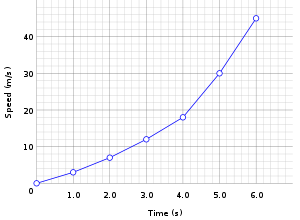## Terms and Terminology Relating to Explaining the Relationship Between Two Variables

• Variable: An amount, quantity or number that can vary and change
• An independent variable: A factor that has some influence or impact on the dependent variable
• Dependent variable: The factor that changes as a result of the influence of the independent variable
• Response variable: Alternative term for dependent variable
• Control variable: The thing or things that are controlled and constant so that they do not impact on the dependent variable
• Covariance: The relationship between two variables
• Positive correlation: When both the independent and dependent variables increase together and at the same time and when both the independent and dependent variables decrease together and at the same time
• Negative correlation: When one of the variables increases at the same time that the other variable decreases

## Variables

These three basic types of variables are:

1. Independent variables
2. Dependent variables
3. Extraneous variables

### Independent Variables

An independent variable is the factor that has some influence or impact on the dependent variable.

### Dependent Variables

A dependent variable is the factor that changes as a result of the influence of the independent variable. It is the behavior, outcome or characteristic that changes as a response to some manipulation. For this reason, a dependent variable is sometimes referred to as a response variable.

Below are some examples of independent and dependent variables:

• Knowledge levels (dependent variable) after an educational class on stress management techniques (independent variable)
• The levels of stress (dependent variable) relating to the increasing severity of a chronic, progressive disease or illness (independent variable)

### Extraneous Variables

Extraneous variables, which are also called, interfering variables, are conditions that undesirably impact on the dependent variable. This undesirable impact on a dependent variable should be prevented with the elimination and/or minimization of all extraneous variables.When variables are put on a graph, the independent variable, like time in the graph above, is typically shown along the horizontal x axis of the graph and the dependent variable, like speed which increases with time in the graph above is typically placed and shown along the y axis.

RELATED TEAS MEASUREMENT & DATA CONTENT:Latest posts by Alene Burke, RN, MSN (see all)